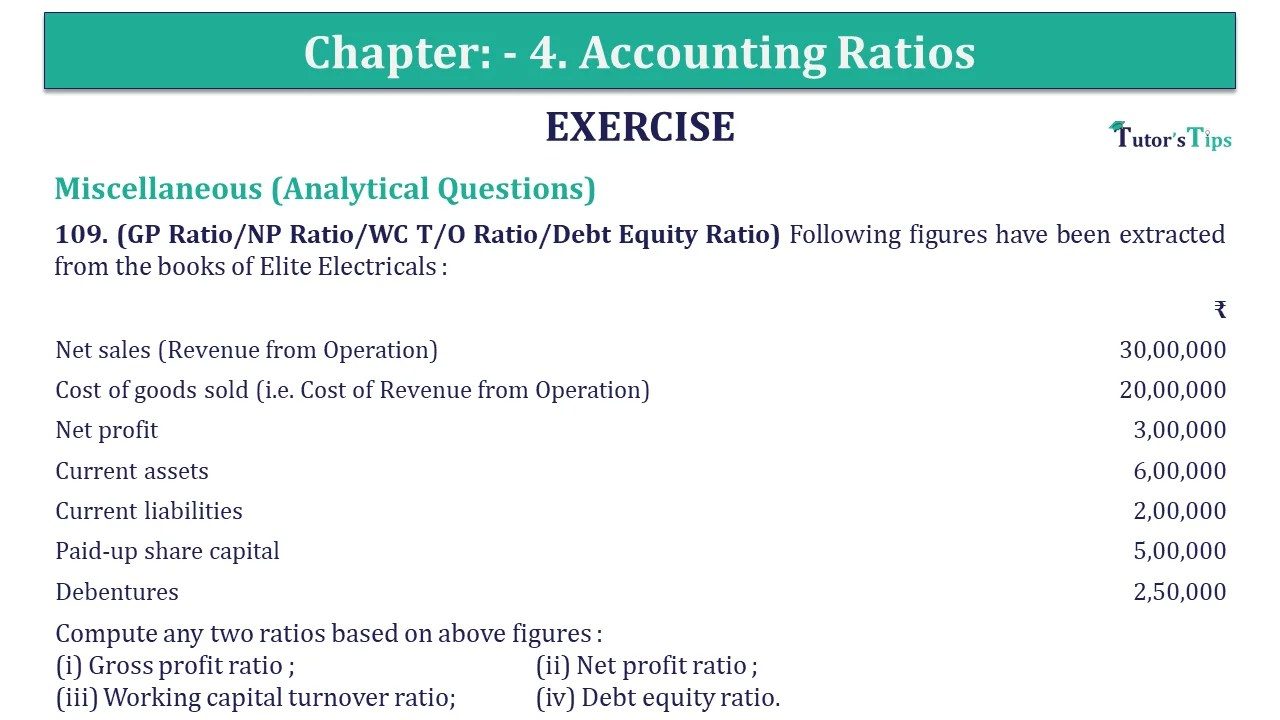# Question 109 Chapter 4 of +2-B – USHA Publication 12 ClassQ-109- CH-4 Book 2 - Usha Pub. +2 Book 2020 - Solution

Question 109 Chapter 4 of +2-B

Miscellaneous (Analytical Questions)

109. (GP Ratio/NP Ratio/WC T/O Ratio/Debt Equity Ratio) Following figures have been extracted from the books of Elite Electricals :

 ₹ Net sales (Revenue from Operation) 30,00,000 Cost of goods sold (i.e. Cost of Revenue from Operation) 20,00,000 Net profit 3,00,000 Current assets 6,00,000 Current liabilities 2,00,000 Paid-up share capital 5,00,000 Debentures 2,50,000

Compute any two ratios based on above figures :
(i) Gross profit ratio ; (ii) Net profit ratio ; (iii) Working capital turnover ratio; (iv) Debt equity ratio.

## The solution of Question 109 Chapter 4 of +2-B: –

 (i) Gross Profit Ratio = Gross Profit X 100 Net Sales = ₹ 10,00,000 X 100 ₹ 30,00,000 = 33.33%
 (ii) Net Profit Ratio = Net Profit X 100 Net Sales = ₹ 3,00,000 X 100 ₹ 30,00,000 = 10%

 (iii) Working Capital Turnover Ratio = Net Sales Net Working Capital = ₹ 30,00,000 ₹ 4,00,000 = 7.5 times
 (iv) Debt Equity Ratio = Debt Equity = ₹ 2,50,000 ₹ 8,00,000 = 2.5: 8

Working Notes :

 Gross Profit = Sales – Cost of goods sold = ₹ 30,00,000 – ₹ 20,00,000 = ₹ 10,00,000 Working Capital = Current Assets – Current Liabilities = ₹ 6,00,000 – ₹ 2,00,000 = ₹ 4,00,000 Shareholders’ Funds or Equity = Equity Share Capital + Net Profit = ₹ 5,00,000 + ₹ 3,00,000 = ₹ 8,00,000

Also, Check out the solved question of previous Chapters: –